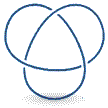Next: How do we work Up: text_knot Previous: Definitions

# The Central Problem of Knot TheoryThe central problem of Knot Theory is determining whether two knots can be rearranged (without cutting) to be exactly alike.

A special case of this problem is one of the fundamental questions of Knot Theory: Given a knot, is it the unknot? Now, for a simple loop, that's an easy question. But, take for example the trefoil knot animated at the top of the page. Is it possible to transform this knot so that it looks like the unknot? Tie a trefoil knot yourself and see if you can untangle it to form a simple circular loop.

When we actually start trying to untangle and rearrange knots to look like one another, we begin what can seem like a very complicated process. Mathematicians were perplexed at the seemingly unending number of ways a knot could be shaped and turned. What was needed was a simple set of rules for working with knots.

Finally, German mathematician Kurt Reidemeister (1893-1971) proved that all the different transformations on knots could be described in terms of three simple moves. The next section will give us the simple tools we need to begin working with knots in a mathematical context.Next: How do we work Up: text_knot Previous: Definitions
Supriya Garg 2004-05-02